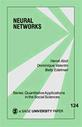Little Green Books

# Neural Networks

Neural networks, adaptive statistical models based on an analogy with the structure of the brain, can be used to estimate the parameters of some population using one (or a few) exemplars at a time. This book introduces readers to the basic models of neural networks and compares and contrasts these models using other statistical models. Through the use of examples that can be computed by hand or with a simple calculator, the authors describe and explain the various models.

locked icon

•••